# Legend with numpoints = 1

Hello,

I would like to make a plot showing data points and a line, where the
legend contains a single point for the data points, and a small line
segment for the line. However, with numpoints = 1, the line is not
shown, as can be seen in the attached figure numpoints1.png. The second
attached figure, desired_legend.png, shows how I would like the legend
to look. I have also attached a simple script that demonstrates the
problem with numpoints=1.

Is there any way to create the legend as I would like it?

Thank you,

Paul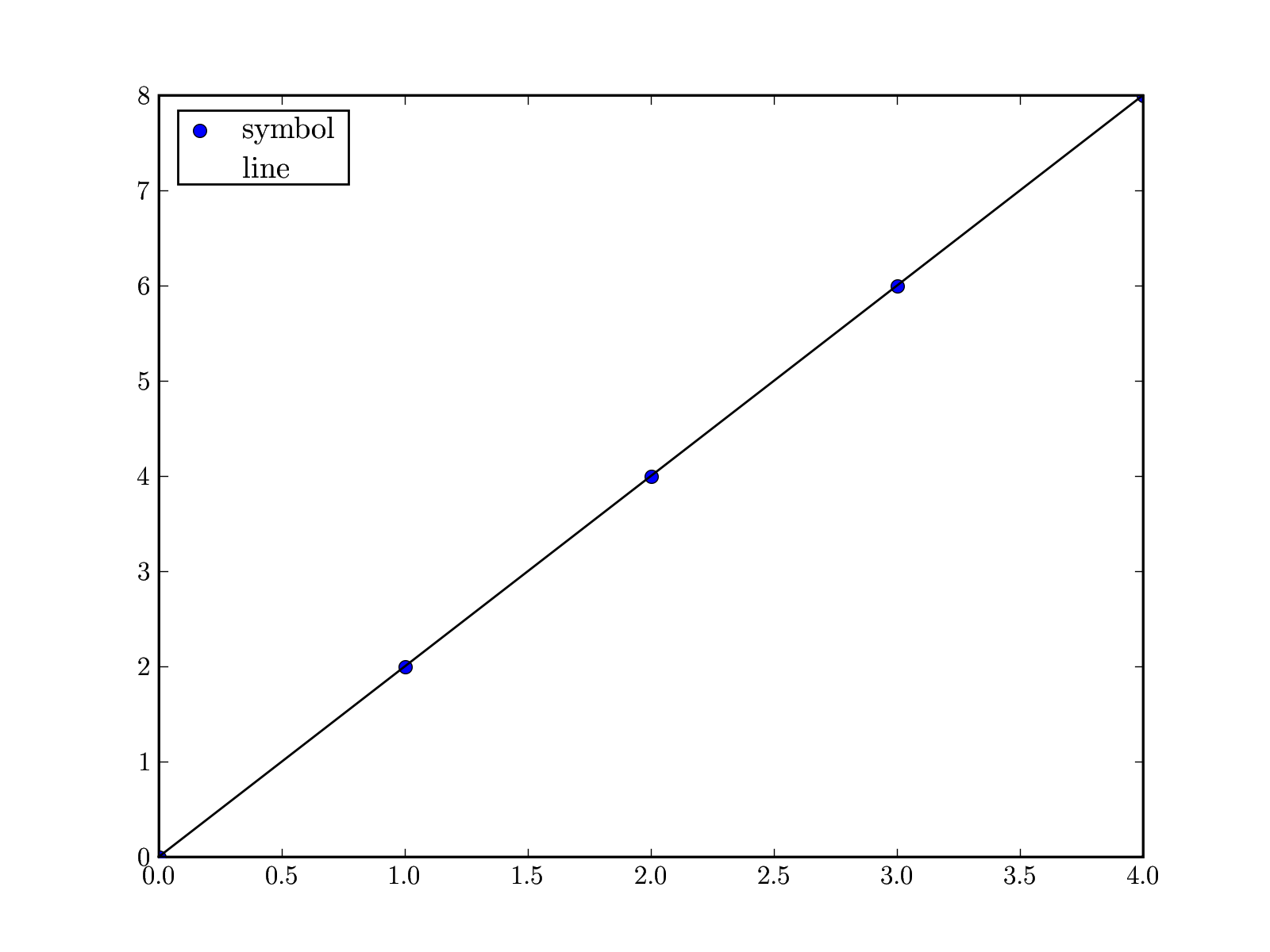legend_test.py (238 Bytes)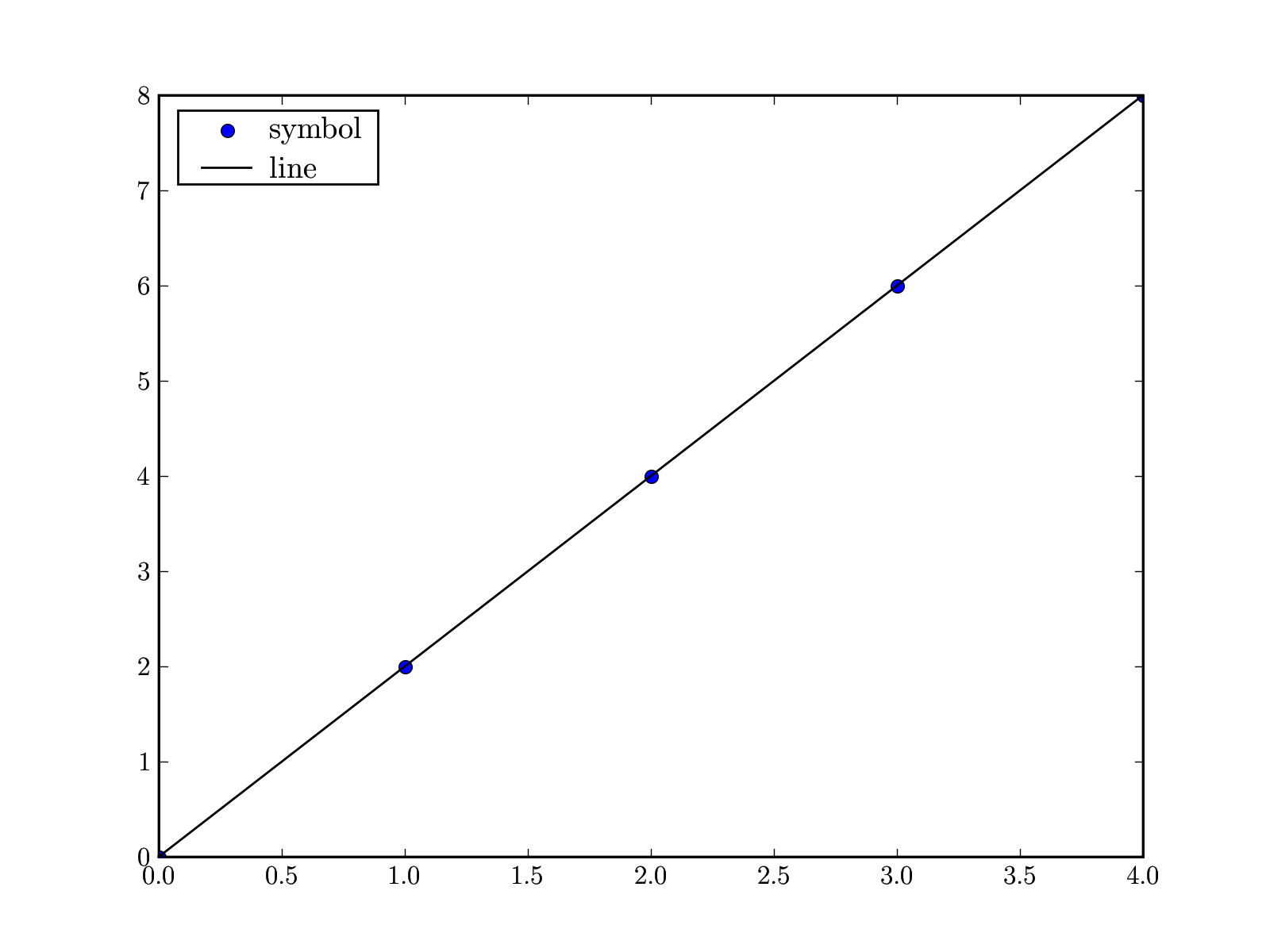Hello,

I have further investigated problems with legend() when numpoints = 1.
The images show what happens when numpoints = 1 for a Line2D, such as
when calling plot(), for a LineCollection, and for a
RegularPolyCollection, such as when using scatter(). As can be seen in
the figures, calling legend() with numpoints = 1 results in either an
absence of a line or a misplacement of a symbol or colored region,
creating an ugly legend.

I have made the three figures using the script included below,
line_collection.py from the examples, and scatter_demo.py from the examples.

I will send a second message that includes a patch that attempts to fix
the problems with legend() when numpoints=1, and some figures showing
the improvement in the legends.

Paul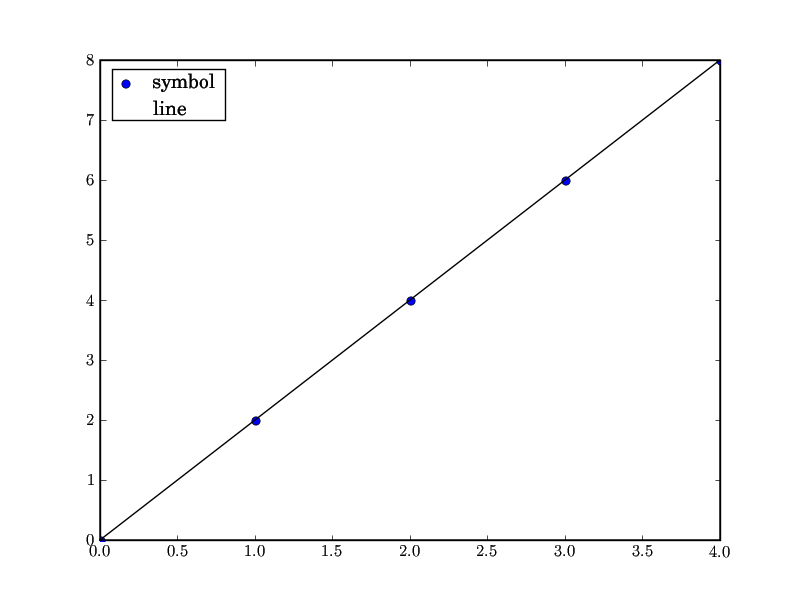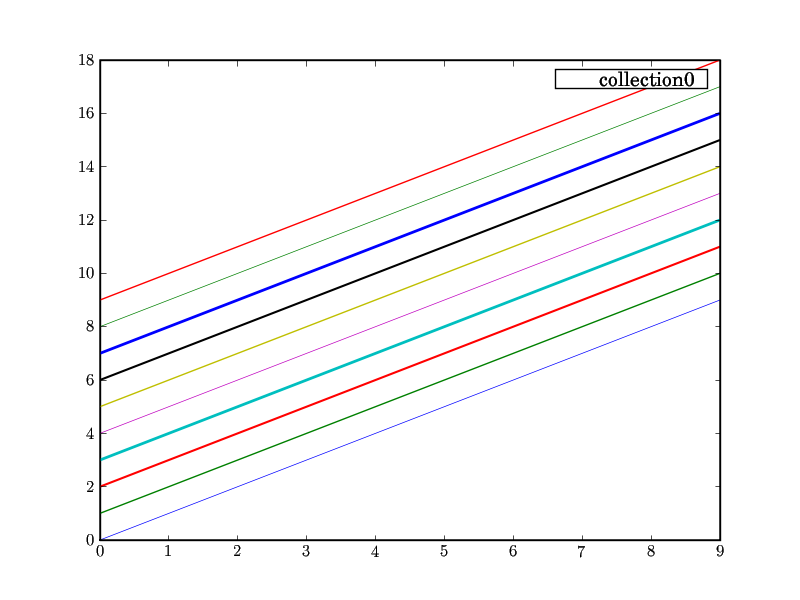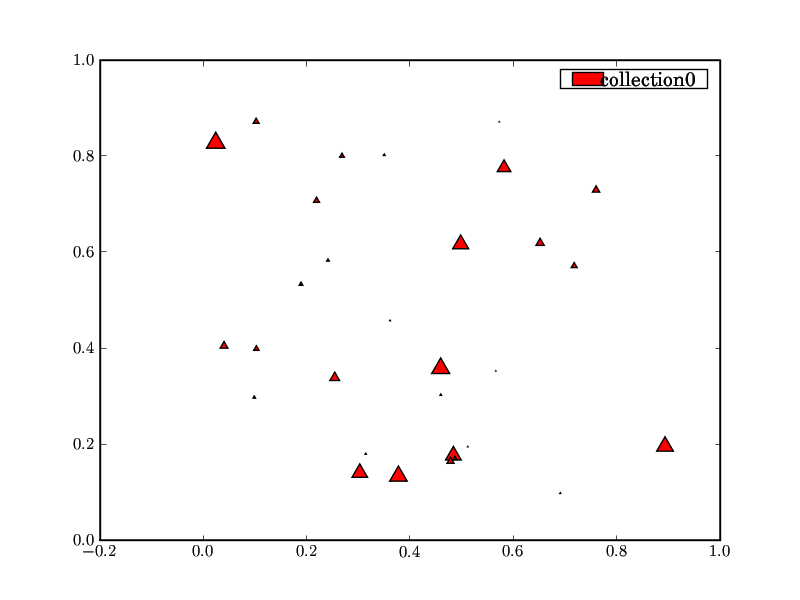···

---
#!/usr/bin/env python

import matplotlib
matplotlib.use('GTKAgg')

from pylab import *

x = arange(0.0, 5.0)
y = 2.0 * x

figure(1)
plot(x, y, 'o', label='symbol')
plot(x, y, 'k-', label='line')

leg=legend(loc='best',numpoints=1)

show()

Hello,

I have included below a patch that attempts to fix the problems with
legend() when numpoints = 1. When numpoints = 1 and a line is plotted,
_marker == 'None', and a line segment of length handlelen is placed in
the legend; when symbols are being plotted, a single symbol is centered
in the handlelen region and placed in the legend. For LineCollection and
RegularPolyCollection, the legend when numpoints = 1 looks like the
legend when numpoints > 1; the legend always has a line or colored
region and the line or colored region and text do not overlap. The
results of the patch can be seen in the included figures.

There are some problems with the patch.
1) The variable left has to be introduced into the function
_get_handles() to properly place the legend markers.
2) Legends which contain a Patch are not handled, because I do not know
which kind of plot would result in a Patch in the legend, so I could not
test the effect of changing the legend.
3) The patch was created against 0.91.1; however, I do not think that
there is any difference in legend.py between 0.91.1 and SVN.

I would appreciate any comments or improvements on the patch.

Thanks,

Paul

diff -u a/lib/matplotlib/legend.py b/lib/matplotlib/legend.py
--- a/lib/matplotlib/legend.py 2008-01-02 16:33:47.000000000 -0600
+++ b/lib/matplotlib/legend.py 2008-01-03 08:56:01.000000000 -0600
@@ -273,6 +273,7 @@

def _get_handles(self, handles, texts):
HEIGHT = self._approx_text_height()
+ left = 0.5

ret = [] # the returned legend lines

@@ -280,6 +281,10 @@
x, y = label.get_position()
x -= self.handlelen + self.handletextsep
if isinstance(handle, Line2D):
+ if self.numpoints == 1 and handle._marker == 'None':
+ self._xdata = npy.linspace(left, left +
self.handlelen, 2)
+ elif self.numpoints == 1:
+ self._xdata = npy.array([left + self.handlelen*0.5])
ydata = (y-HEIGHT/2)*npy.ones(self._xdata.shape, float)
legline = Line2D(self._xdata, ydata)
legline.update_from(handle)
@@ -298,6 +303,8 @@
p.set_clip_box(None)
ret.append(p)
elif isinstance(handle, LineCollection):
+ if self.numpoints == 1:
+ self._xdata = npy.linspace(left, left +
self.handlelen, 2)
ydata = (y-HEIGHT/2)*npy.ones(self._xdata.shape, float)
legline = Line2D(self._xdata, ydata)
self._set_artist_props(legline)
@@ -311,6 +318,8 @@
ret.append(legline)

elif isinstance(handle, RegularPolyCollection):
+ if self.numpoints == 1:
+ self._xdata = npy.array([left])
p = Rectangle(xy=(min(self._xdata), y-3/4*HEIGHT),
width = self.handlelen, height=HEIGHT/2,
)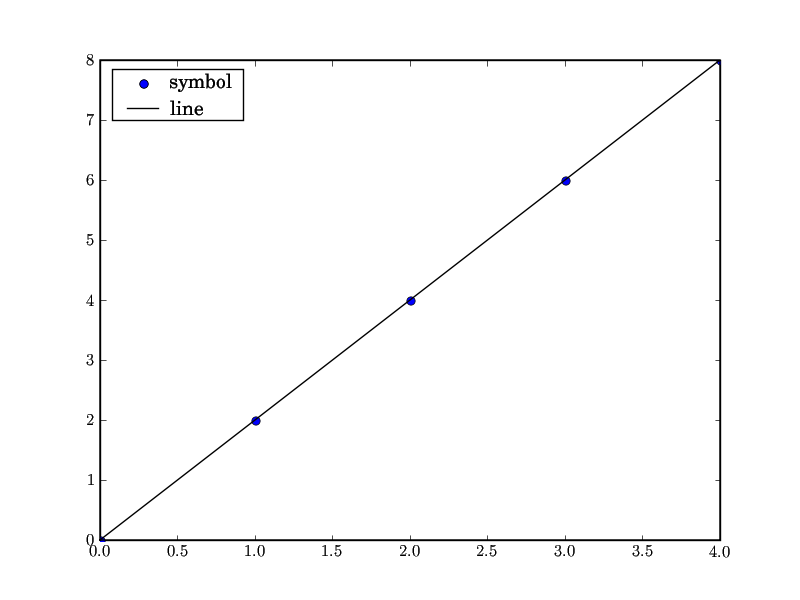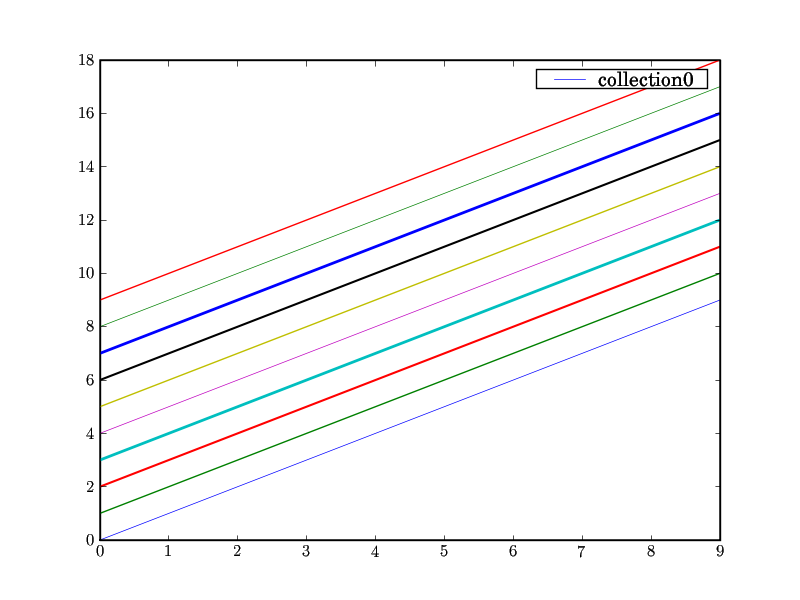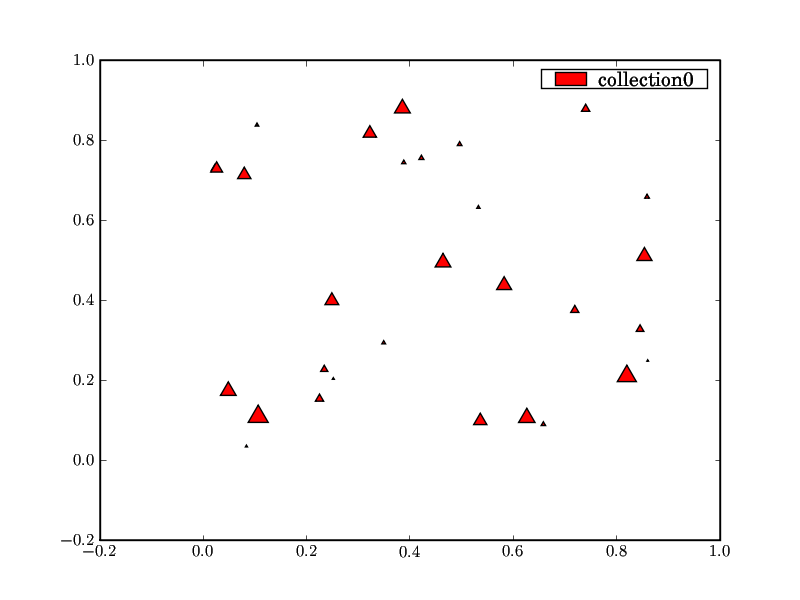I'm sure the radio silence to your question is just due to holidays.

Thanks for looking into this. I'd be happy to incorporate your patch when it is ready.

As for your question about plots that can include patches -- patches are virtually anything plotted that aren't lines or images. This includes rectangles, polygons and ellipses, for instance. See something like ellipse_demo.py for an example. Patches are always drawn as rectangles in the legend.

Cheers,
Mike

Paul Novak wrote:

···

Hello,

I have further investigated problems with legend() when numpoints = 1.
The images show what happens when numpoints = 1 for a Line2D, such as
when calling plot(), for a LineCollection, and for a
RegularPolyCollection, such as when using scatter(). As can be seen in
the figures, calling legend() with numpoints = 1 results in either an
absence of a line or a misplacement of a symbol or colored region,
creating an ugly legend.

I have made the three figures using the script included below,
line_collection.py from the examples, and scatter_demo.py from the examples.

I will send a second message that includes a patch that attempts to fix
the problems with legend() when numpoints=1, and some figures showing
the improvement in the legends.

Paul

---
#!/usr/bin/env python

import matplotlib
matplotlib.use('GTKAgg')

from pylab import *

x = arange(0.0, 5.0)
y = 2.0 * x

figure(1)
plot(x, y, 'o', label='symbol')
plot(x, y, 'k-', label='line')

leg=legend(loc='best',numpoints=1)

show()

------------------------------------------------------------------------

------------------------------------------------------------------------

------------------------------------------------------------------------

------------------------------------------------------------------------

-------------------------------------------------------------------------
This SF.net email is sponsored by: Microsoft
Defy all challenges. Microsoft(R) Visual Studio 2005.
http://clk.atdmt.com/MRT/go/vse0120000070mrt/direct/01/

------------------------------------------------------------------------

_______________________________________________
Matplotlib-users mailing list
Matplotlib-users@lists.sourceforge.net
https://lists.sourceforge.net/lists/listinfo/matplotlib-users

--
Michael Droettboom
Science Software Branch
Operations and Engineering Division
Space Telescope Science Institute
Operated by AURA for NASA

Included below is an updated patch fixing the legend with numpoints=1. The patch has been made against SVN and works for Line2D, LineCollection, Patch, and RegularPolyCollection. The patch could be merged.

I also think the patch could be improved. Currently, handle._marker is examined to determine if the legend should contain lines or symbols, but this is done in the _get_handles function. It would be better if that could be moved into the Legend class definition, however, I do not know how to examine handle._marker in the Legend class definition.

Again, I would appreciate any comments or improvements.

Thanks,

Paul

diff -u a/lib/matplotlib/legend.py b/lib/matplotlib/legend.py
--- a/lib/matplotlib/legend.py 2008-01-09 13:11:00.000000000 -0600
+++ b/lib/matplotlib/legend.py 2008-01-09 13:08:36.000000000 -0600
@@ -175,9 +175,7 @@
# make a trial box in the middle of the axes. relocate it
# based on it's bbox
left, top = 0.5, 0.5
- if self.numpoints == 1:
- self._xdata = npy.array([left + self.handlelen*0.5])
- else:
+ if self.numpoints > 1:
self._xdata = npy.linspace(left, left + self.handlelen, self.numpoints)
textleft = left+ self.handlelen+self.handletextsep
self.texts = self._get_texts(labels, textleft, top)
@@ -236,6 +234,7 @@

def _get_handles(self, handles, texts):
HEIGHT = self._approx_text_height()
+ left = 0.5

ret = [] # the returned legend lines

@@ -243,6 +242,10 @@
x, y = label.get_position()
x -= self.handlelen + self.handletextsep
if isinstance(handle, Line2D):
+ if self.numpoints == 1 and handle._marker == 'None':
+ self._xdata = npy.linspace(left, left + self.handlelen, 2)
+ elif self.numpoints == 1:
+ self._xdata = npy.array([left + self.handlelen*0.5])
ydata = (y-HEIGHT/2)*npy.ones(self._xdata.shape, float)
legline = Line2D(self._xdata, ydata)
legline.update_from(handle)
@@ -253,7 +256,8 @@

ret.append(legline)
elif isinstance(handle, Patch):

···

-
+ if self.numpoints == 1:
+ self._xdata = npy.linspace(left, left + self.handlelen, 2)
p = Rectangle(xy=(min(self._xdata), y-3/4*HEIGHT),
width = self.handlelen, height=HEIGHT/2,
)
@@ -263,6 +267,8 @@
p.set_clip_path(None)
ret.append(p)
elif isinstance(handle, LineCollection):
+ if self.numpoints == 1:
+ self._xdata = npy.linspace(left, left + self.handlelen, 2)
ydata = (y-HEIGHT/2)*npy.ones(self._xdata.shape, float)
legline = Line2D(self._xdata, ydata)
self._set_artist_props(legline)
@@ -277,6 +283,8 @@
ret.append(legline)

elif isinstance(handle, RegularPolyCollection):
+ if self.numpoints == 1:
+ self._xdata = npy.array([left])
p = Rectangle(xy=(min(self._xdata), y-3/4*HEIGHT),
width = self.handlelen, height=HEIGHT/2,
)

Michael Droettboom wrote:

I'm sure the radio silence to your question is just due to holidays.

Thanks for looking into this. I'd be happy to incorporate your patch when it is ready.

As for your question about plots that can include patches -- patches are virtually anything plotted that aren't lines or images. This includes rectangles, polygons and ellipses, for instance. See something like ellipse_demo.py for an example. Patches are always drawn as rectangles in the legend.

Cheers,
Mike